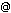prof. RNDr.JiříRosický, DrSc.

učo 2634
rosickymailmunicz rosickymathmunicz
Phone: 549 49 6758

Department

Position Department of Mathematics and Statistics - Departments - Faculty of Science professor 02011 (Kotlářská 2, Brno — Pav.8, 1.p.) 549 49 6758

Membership in academic institutions

Faculty of Science
Doctoral Board (programme Matematics (4-years))
Oborová rada (programme Matematika a statistika)
Oborová rada (programme Mathematics and Statistics)
PhD supervisors (fields of study Algebra, Algebra, Number Theory and Mathematical Logic and Algebra, Number Theory and Mathematical Logic (Eng.))
Doctoral Committee (fields of study Algebra, Number Theory and Mathematical Logic, Algebra, Number Theory and Mathematical Logic (Eng.), Algebra, Number Theory and Mathematical Logic and Algebra, Number Theory and Mathematical Logic (Eng.))
Školitelé Ph.D. (programme Mathematics and Statistics)